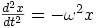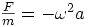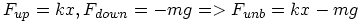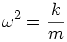Warning: Illegal string offset 'html' in /home/hsn/public_html/forum/cache/skin_cache/cacheid_1/skin_topic.php on line 909

2003 Q4 - HSN forum# 2003 Q4

3 replies to this topic

### #1679810

• Gender:Male

Posted 15 January 2007 - 06:33 PM

I bet it's really easy and I'll kick myself for not knowing it but any chance of some help

A mass of 1.5kg is suspended from a spring

This extends the length of the spring from 40mm to 80 mm. The mass is at rest

(a)Calculate the force exerted by the spring on the mass

### #2679810

• Gender:Male

Posted 15 January 2007 - 07:02 PM

I bet it's really easy and I'll kick myself for not knowing it but any chance of some help

A mass of 1.5kg is suspended from a spring

This extends the length of the spring from 40mm to 80 mm. The mass is at rest

(a)Calculate the force exerted by the spring on the mass

Yup I was just looking at the wrong answer so turns out I got it right

Next question:D

(4c)The mass is now pulled down, extending the spring a further 30mm

The mass is released and the subsequent motion is simple harmonic
The force exerted by the spring is directly proportional to its extension

(i)Calculate the unbalanced force acting on the mass just after its release
(ii)Calculate the period of oscillation of the mass

Top of the Class

•• Members
•• 390 posts
• Location:Cambridge
• Interests:Muzak.
• Gender:Male

Posted 16 January 2007 - 06:10 PM

i)The force exerted by the spring is directly proportional to the extension of the spring. So T=kl, where T is the tension in the spring, l is the extension, and k is the constant of proportionality. Use part (a) to find k, then use this value of k to find the tension in the spring for the new extension.

ii)(from part (i), you calculated the force acting at the extremity. Let a be the amplitude of the SHM, and F be the unbalanced force you calculated in (i) - this is just the SHM equation written at the extremity)Solve for T
HMFC - Founded 1874, beefing the Cabbage since 1875

### #4waitingforan_alibi

• Gender:Male

Posted 19 January 2007 - 08:41 PM

I bet it's really easy and I'll kick myself for not knowing it but any chance of some help

A mass of 1.5kg is suspended from a spring

This extends the length of the spring from 40mm to 80 mm. The mass is at rest

(a)Calculate the force exerted by the spring on the mass

Yup I was just looking at the wrong answer so turns out I got it right

Next question:D

(4c)The mass is now pulled down, extending the spring a further 30mm

The mass is released and the subsequent motion is simple harmonic
The force exerted by the spring is directly proportional to its extension

(i)Calculate the unbalanced force acting on the mass just after its release
(ii)Calculate the period of oscillation of the mass

(i)(ii)equate and solve for T

#### 1 user(s) are reading this topic

0 members, 1 guests, 0 anonymous users